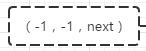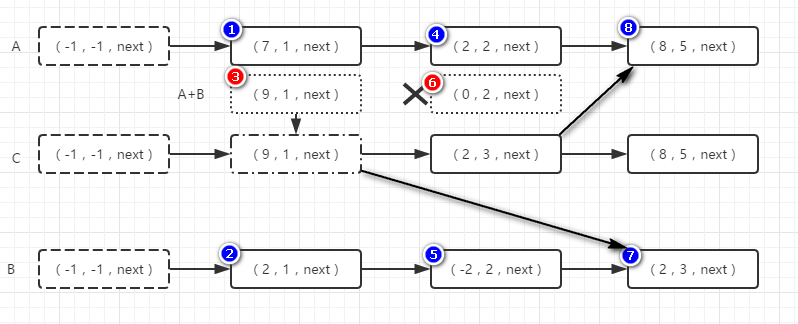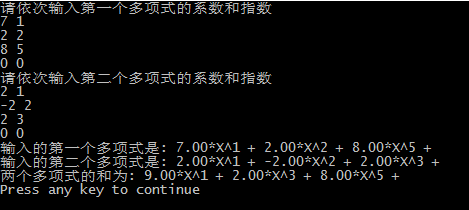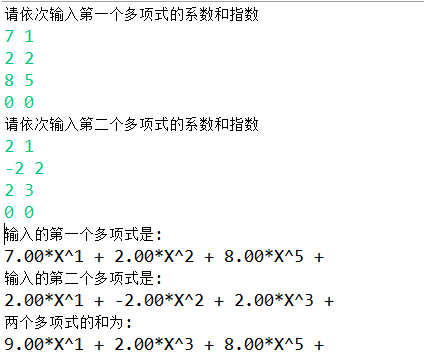# 链表实现两个多项式的加法

#### 一、题目要求

多项式A: X^1 + 2 * X^2 + 3 * X^3

1 1
2 2
3 3
0 0
1 1
2 2
3 3
0 0

2 * X^1 + 4 * X^2 + 6 * X^3

#### 二、题目分析链表A表示的多项式: 7 * X^1 + 2 * X^2 + 8 * X^5

1 . 我们规定三个头指针,分别指向三个链表的头,然后再规定三个移动指针,分别指向当前三个链表中正在处理的那个节点

2 . 我们让A、B、C的移动指针刚开始也处于头指针的位置,然后,我们拿A第一个节点中的指数和B第一个节点中的指数进行比较,这个时候有三种情况:

a情况 . A中当前的节点指数 < B中当前的节点指数 —— 我们将A中的当前节点插入C中,然后向后移动A和C的指针(因为A中当前节点已经处理了)

b情况 . A中当前的节点指数 > B中当前的节点指数 —— 我们将B中的当前节点插入C中,然后向后移动B和C的指针(因为B中当前节点已经处理了) 即图中⑧的情况。

c情况 . A中当前的节点指数 > B中当前的节点指数 —— 此时A和B当前节点指数相同,可以进行系数相加,这时候也会出现两种情况:

#### 三、代码实现

C语言实现:

#include<stdio.h>
#include<malloc.h>
typedef struct node{
float coef;
int expn;
node *next;
}Lnode, * Dxs;

Dxs create();
void printDxs(Dxs h);
void deleteNode(Dxs h, Dxs p);

int main(){
Dxs ha, hb, hc;
printf("请依次输入第一个多项式的系数和指数\n");
ha = create();
printf("请依次输入第二个多项式的系数和指数\n");
hb = create();
printf("输入的第一个多项式是: ");
printDxs(ha->next);
printf("输入的第二个多项式是: ");
printDxs(hb->next);
printf("两个多项式的和为: ");
printDxs(hc->next);
return 0;
}

//创建链表(读入数据以 0 0 结束)
Dxs create(){
float coef;
int expn;
Dxs first, qa, s;
first = (Dxs)malloc(sizeof(Lnode));
first->coef = -1;
first->expn = -1;
first->next = NULL;
qa = first;
while(1){
scanf("%f", &coef);
scanf("%d", &expn);
if(coef == 0 && expn == 0){
break;
}
s = (Dxs)malloc(sizeof(Lnode));
s->coef = coef;
s->expn = expn;
s->next = NULL;
qa->next = s;
qa = s;
}
return first;
}

//链表相加
Dxs firstc, ha, hb, pc, s;
int a, b;
float sum;
firstc = (Dxs)malloc(sizeof(Lnode));
firstc->coef = -1;
firstc->expn = -1;
firstc->next = NULL;
pc = firstc;

ha = firsta->next;
hb = firstb->next;
while(ha!= NULL && hb != NULL){
a = ha->expn;
b = hb->expn;
if(a < b){
//将a加入c中,移动a和c的指针
pc->next = ha;
pc = pc->next;
ha = ha->next;
}else if(a > b){
//将b加入c中,移动b和c的指针
pc->next = hb;
pc = pc->next;
hb = hb->next;
}else{
sum = ha->coef + hb->coef;
if(sum != 0.0){
//将和加入a中,再将a加入c中,移动c的指针
ha->coef = sum;
pc->next = ha;
pc = pc->next;
}else{

//查找删除A中系数之和为0的那个节点
s = firsta;
while(s != ha){
s = s->next;
}
s->next = ha->next;
}
//ab已经处理完成,同时后移一位
ha = ha->next;
hb = hb->next;
}
}

//将剩余部分加入c后面
if(ha != NULL){
pc->next = ha;
}

if(hb != NULL){
pc->next = hb;
}
return firstc;
}

//遍历显示链表
void printDxs(Dxs h){
while(h != NULL){
printf("%0.2f*X^%d + ", h->coef, h->expn);
h=h->next;
}
printf("\n");
}

Java语言描述:

package cn.codekong;

import java.util.Scanner;

/**
* 链表类
* @author szh
*
*/

/**
* 链表节点类
* @author szh
*
*/
class Node{
//多项式的系数
private float coef;
//多项式的指数
private int expn;
//指向下级节点
public Node next = null;
public Node(float coef, int expn){
this.coef = coef;
this.expn = expn;
}
}

/**
* 创建链表类
* 从从控制台不断读入数据，以(0 0)结束
* @return  创建好链表的第一个节点
*/
//存取从控制台读到的系数和指数
float coef = 0.0f;
int expn = 0;
//头尾节点(尾节点方便插入)
Scanner scanner = new Scanner(System.in);
while(true){
String res = scanner.nextLine();
//以空格分割一行中的字符串
String[] resArray = res.split("\\s+");
coef = Float.parseFloat(resArray);
expn = Integer.parseInt(resArray);
if(coef == 0 && expn == 0.0f){
break;
}
Node node = new Node(coef, expn);
node.next = null;
tail.next = node;
tail = tail.next;
}
}

/**
* 打印链表
*/
}
System.out.println();
}

/**
* 计算两个链表的和
* @param nodeA
* @param nodeB
* @return  最终和的链表
*/
Node nodeC = new Node(-1, -1);
nodeC.next = null;
//始终指向链表的当前需要处理的节点(刚开始要除去开头的(-1,-1)节点)
Node pA = nodeA.next, pB = nodeB.next, pC = nodeC;
//当前指向的两个链表的指数
int valueAExpn = 0, valueBExpn = 0;
while(pA != null && pB != null){
valueAExpn = pA.expn;
valueBExpn = pB.expn;
if(valueAExpn < valueBExpn){
//将A中的该节点加入C中, 同时移动A和C的指针指向下个元素
pC.next = pA;
pC = pC.next;
pA = pA.next;
}else if(valueAExpn > valueBExpn){
//将B中的该节点加入C中,同时移动B和C的指针指向下个元素
pC.next = pB;
pC = pC.next;
pB = pB.next;
}else{
//两节点指数相同
//现将系数相加放到A节点的系数中,然后将A节点加入C中
float sum = pA.coef + pB.coef;
if(sum != 0.0f){
//系数和不为0,将A节点的系数改变为sum,然后将A节点加入C中
pA.coef = sum;
pC.next = pA;
pC = pC.next;
}else{
//系数和为0,必须将A链表中的该节点从链表中删除掉,如果只移动指针,会在输出时也输出该项
//在整个A链表中依次查找,必须找到要删除节点的前驱节点才能将其删除
Node s = nodeA;
while(s != pA){
s = s.next;
}
//删除该节点
s.next = pA.next;
}
//对于系数相同的情况,A和B节点指针都往后移动
pA = pA.next;
pB = pB.next;
}
}
if(pA != null){
pC.next = pA;
}
if(pB != null){
pC.next = pB;
}
return nodeC;
}
}
package cn.codekong;

public class MyTest {
public static void main(String[] args) {
System.out.println("请依次输入第一个多项式的系数和指数");
System.out.println("请依次输入第二个多项式的系数和指数");
System.out.println("输入的第一个多项式是: ");
System.out.println("输入的第二个多项式是: ");
System.out.println("两个多项式的和为: ");
}
}

#### 四、代码运行验证

C语言运行结果：Java运行结果：